# Percent to Fraction Calculator

%

## Convert percent value into simple fraction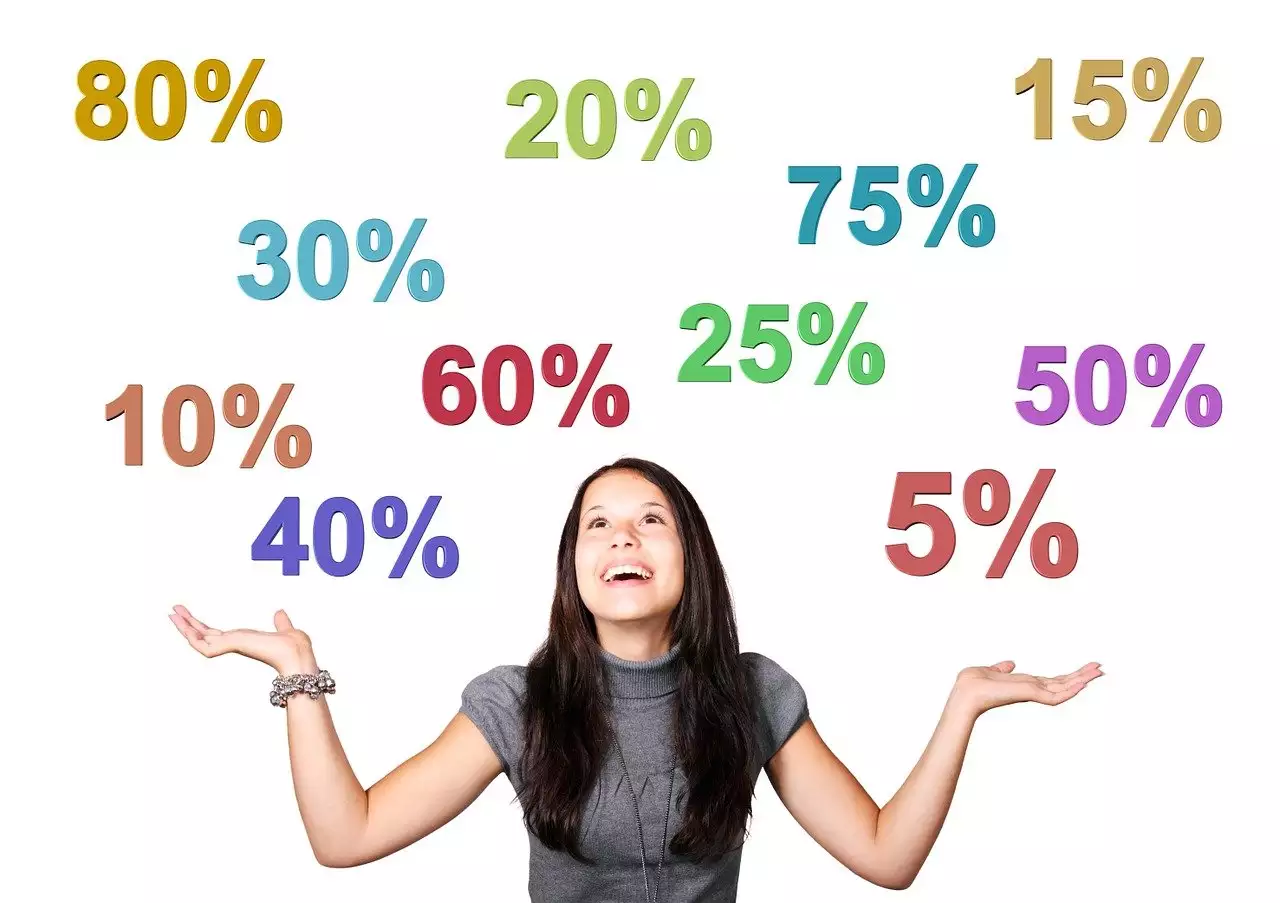This percent-to-fraction calculator is an online tool designed by Calcopolis to help you convert any percent to a fraction quickly and accurately. You can even enter percentages with decimal places and have them turned into fractions.

You can also revert such an operation using a fraction to percent converter.

It's worth noting that if you convert a percent with a value greater than 100%, you'll get a mixed number rather than a fraction.

To explain, any percentage greater than 100% results in improper fractions. This is a type of fraction in which the numerator (top number) is greater in value than the denominator (bottom number), such as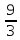.

Improper fractions are usually converted to mixed numbers via long division, which this online calculator does automatically.

## Why Is It So Convenient to Convert Percent to Fractions?

Since we use fractions in diverse aspects of our lives, converting percents to fractions can be useful in a variety of scenarios.

You see, fractions represent a part of a whole or simply something bigger. Thus, they help you better understand what a specific percentage indicates.

For example, suppose you were shopping and came across a pair of socks on sale for 25% off. By converting this percent to a fraction, you'll know that you'll have this item for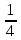off its original price.

## How to Turn a Percent Into a Fraction?

Now, let's look at how to convert a percent to a fraction by hand. This calculation is quite simple and consists of two or three major steps, depending on the percent value.

If it's a whole number, like 60, you only need to follow two steps to convert it to a fraction. If the percent has a decimal place, such as 35.5, you'll go through three steps.

Below are all the steps explained in detail, with an example at the end; take a look:

### Step 1: Divide by 100

The first step is to convert the percent to a whole number or decimal, depending on the case. To accomplish this, divide the percent you have by 100.

For example, if the percent is 38,5%, in this step, you should change it to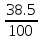, to get 0.385

### Step 2 (Optional): Convert Decimal to Whole Number

This step is only for percents with decimal places; if yours has a whole number, you can skip it. If you have a decimal number, you must convert it to a whole number.

Begin by counting the number of digits to the right of the decimal point. The result x will be the power of the numerator and denominator in a fraction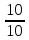. Then, divide this fraction,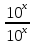, by the decimal number to remove the decimal places.

### Step 3: Simplify the Fraction

In general, any fraction can be reduced if the numerator and denominator can both be divided by the same number.

If you have a fraction that can be simplified, find the Greatest Common Factor (GCF). Then, divide the numerator and denominator by the GCF to get a reduced fraction.

#### Example: Convert 87.5% To a Fraction

1. Divide the percent value by 100: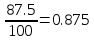2. Since there are three places after the decimal, multiply it by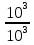: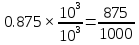3. To simplify this fraction, multiply the numerator and denominator by the GCF, which is 125: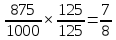## Real-life Applications of Percent to Fraction Conversion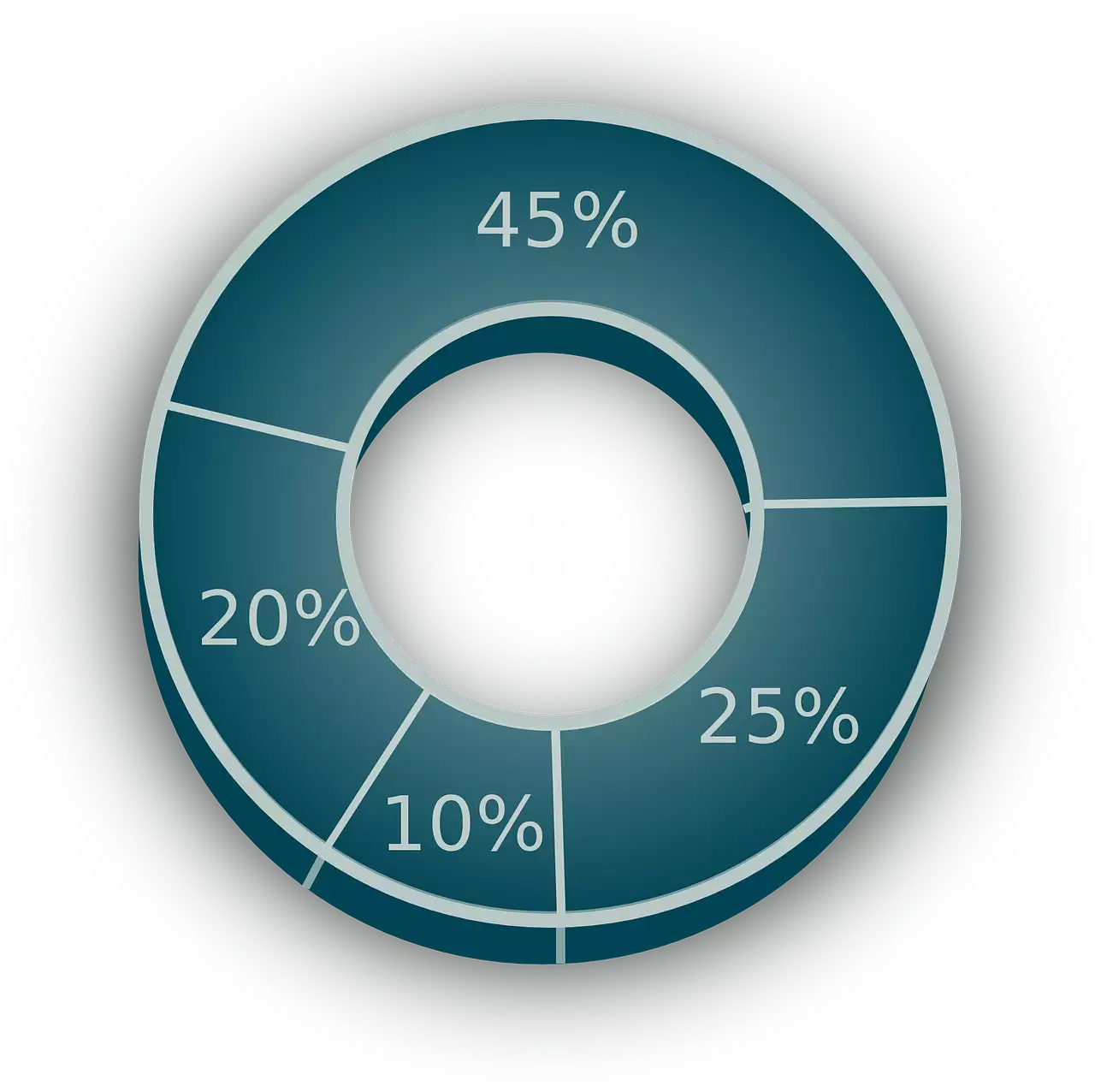Understanding the practical implications of converting percentages to fractions can offer clarity in many professional and academic scenarios. Here's how the percent-to-fraction conversion plays a crucial role in certain areas:

1. Financial Planning: When delineating budget allotments or investments, professionals often convert percentages to simplified fractions. This helps in visualizing the division of funds more clearly, providing insights into how money is distributed or saved.

2. Academics: Students and educators alike benefit from the ability to reduce fractions to their simplest forms. When deciphering complex data or results, converting a percentage and then dividing both the numerator and the denominator can simplify interpretations.

3. Making Reports & Presentations: In corporate settings, executives and managers often deal with data-driven decisions. When presenting this data, converting percentages into fractions can make the information more digestible, especially for stakeholders who prefer fractional representations.

4. Charts & Infographics: Graphic designers and data analysts use percent to fraction conversion to represent information more visually. A bar representing 3/4 might be more impactful and easier to understand than one representing 75%.

5. Sports Analytics: In the realm of sports statistics, coaches and analysts often discuss success rates as percentages. Converting these to fractions offers a tangible perspective on an athlete's performance, helping in strategy formulation.

Professionals and students can seamlessly convert percentages by using our percent to fraction calculator, ensuring the results match the closest simplified fraction.

## Similar tools

This is just one of many handy math tools available at Calcopolis. Our website provides many converters that let you avoid silly mistakes in your routine calculations.

For example, you can find a ratio simplifier or a tool for simplifying complex fractions into more readable versions.

If you wish to master fraction calculations, you may refresh your memory on the concepts you learned at your primary school, like the Greatest Common Factor and Least Common Denominator. It will let you avoid many mistakes in the future.

### Authors

Created by Lucas Krysiak on 2022-12-22 16:53:53 | Last review by Mike Kozminsky on 2022-12-22 18:07:20